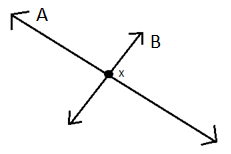QUESTION

# The shortest distance between two intersecting lines is always equal to _________.

Hint: The shortest distance between two lines is equal to determining how far apart lines are. This can be done by measuring the length of a line that is perpendicular to both of them. and in this case, we have two intersecting lines.ax + by + ${{\text{d}}_{\text{1}}}$ = 0 and ax + by + ${{\text{d}}_{\text{2}}}$ = 0
${\text{d = }}\dfrac{{|{d_2} - {d_1}|}}{{\sqrt {{a^2} + {b^2}} }}$ .